## How to vlookup value with multiple criteria in Excel?

In Excel, we can quickly vlookup the corresponding data in a list based on a specific criterion that you set by using the vlookup function. But, if you need to vlookup the relative value based on multiple criteria as below screenshot shown, how could you deal with it?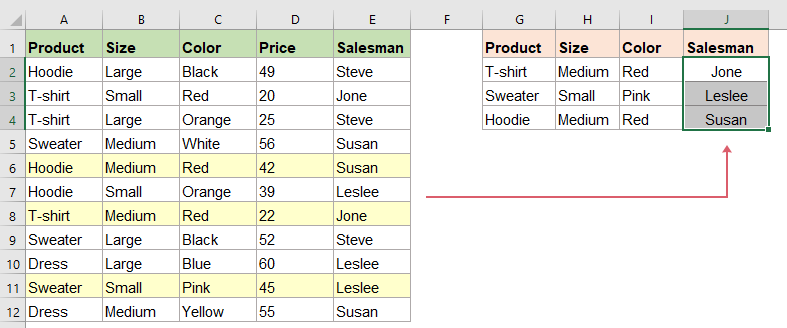Vlookup value with multiple criteria with LOOKUP function

Vlookup value with multiple criteria with INDEXT and MATCH function

Vlookup value with multiple criteria with a useful feature

Supposing I have the following data range which I want to use two criteria to return the relative value, for example, I know the product and color need to return their corresponding saleman in the same row: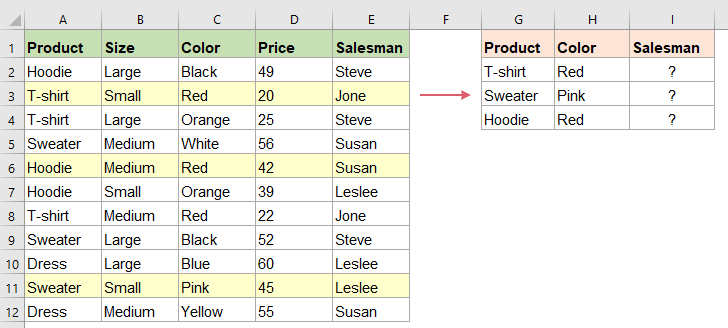#### Vlookup value with multiple criteria with LOOKUP function

LOOKUP function may help you solve this problem, please type this formula into a specified cell, and press Enter key to get the correct result you would like, see screenshot:

=LOOKUP(2,1/(\$A\$2:\$A\$12=G2)/(\$C\$2:\$C\$12=H2),(\$E\$2:\$E\$12))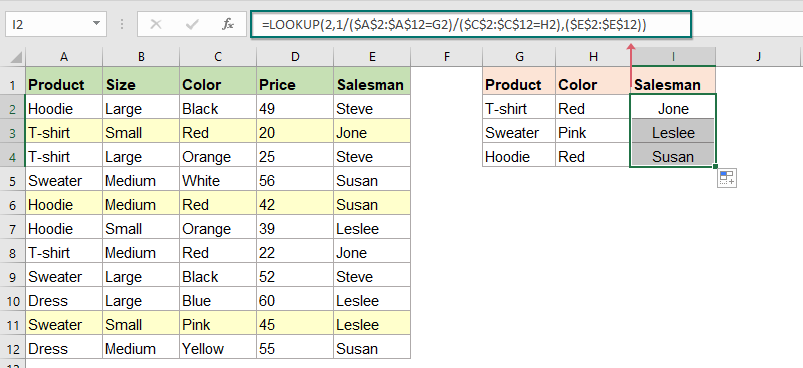Note: In the above formula:

• A2:A12=G2: which means to search the criteria of G2 in range A2:A12;
• C2:C12=H2: means to search the criteria of H2 in range C2:C12;
• E2:E12: refers to the range which you want to return the corresponding value.

Tips: If you have more than two criteria, you just need to add the criteria into the formula as this: =LOOKUP(2,1/(\$A\$2:\$A\$12=G2)/(\$B\$2:\$B\$12=H2)/(\$C\$2:\$C\$12=I2),(\$E\$2:\$E\$12)).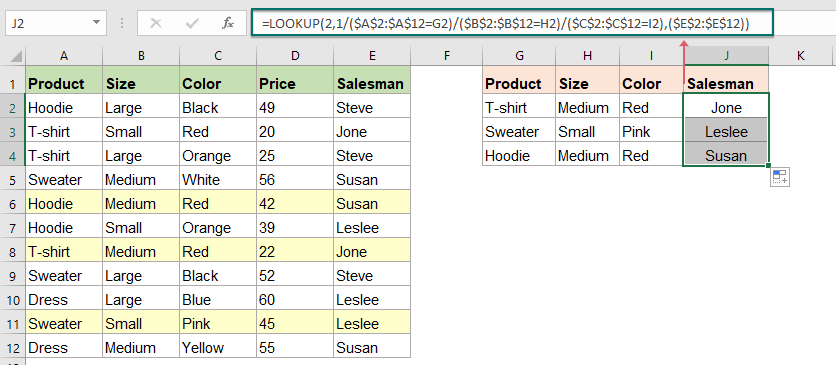#### Vlookup value with multiple criteria with INDEXT and MATCH function

In Excel, the mixed INDEXT and MATCH function is powerful for us to vlookup values based on one or more criteria, to know this formula, do as follows:

Type the below formula into a blank cell, and press Ctrl + Shift + Enter keys together, then you will get the relative value as you want, see screenshot:

=INDEX(\$E\$2:\$E\$12,MATCH(1,(\$A\$2:\$A\$12=G2)*(\$C\$2:\$C\$12=H2),0))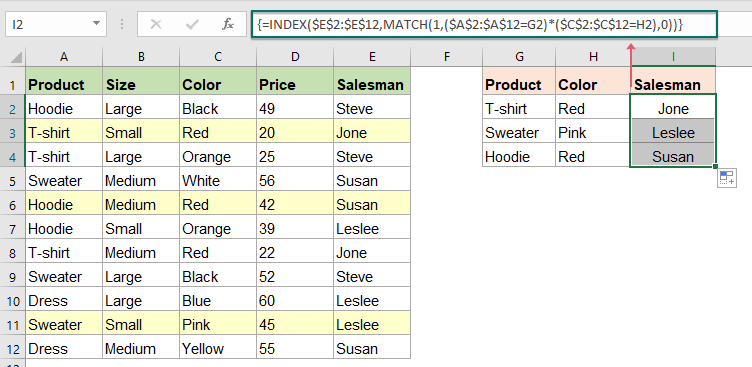Note: In the above formula:

• A2:A12=G2: which means to search the criteria of G2 in range A2:A12;
• C2:C12=H2: means to search the criteria of H2 in range C2:C12;
• E2:E12: refers to the range which you want to return the corresponding value.

Tips: If you have more than two criteria, you just need to add the criteria into the formula as this: =INDEX(\$E\$2:\$E\$12,MATCH(1,(\$A\$2:\$A\$12=G2)*(\$B\$2:\$B\$12=H2)*(\$C\$2:\$C\$12=I2),0)).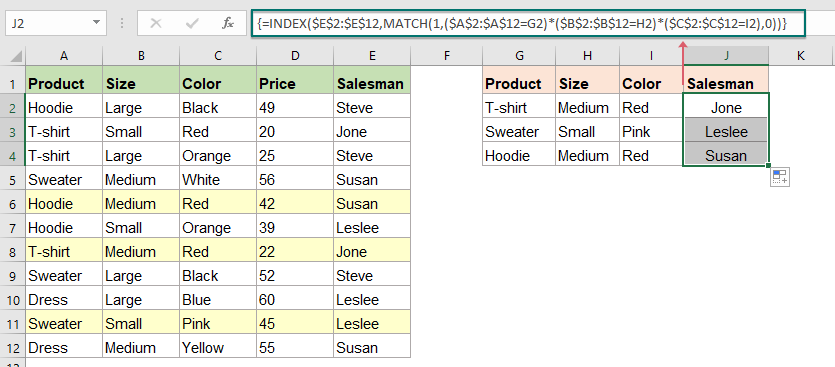#### Vlookup value with multiple criteria with a useful feature

If you have Kutools for Excel, with its Multi-condition Lookup feature, you can quickly return the matching values based on multiple criteria as you need.

Note:To apply this Multi-condition Lookup, firstly, you should download the Kutools for Excel, and then apply the feature quickly and easily.

After installing Kutools for Excel, please do as this:

1. Click Kutools > Super LOOKUP > Multi-conditiion Lookup, see screenshot: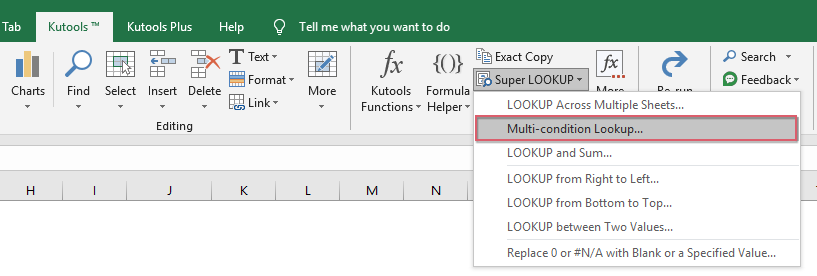2. In the Multi-condition Lookup dialog box, please do the following operations:

• (1.) In the Lookup Values section, specify the lookup value range or select the lookup value column one by one by holding the Ctrl key that you want to vlookup values based on;
• (2.) In the Output Range section, select the output range where you want to put the matching results;
• (3.) In the Key column section, please select the corresponding key columns that contain the lookup values one by one by holding the Ctrl key;
• Note: The number of columns selected in the Key column field must be equal to the number of columns selected in the Lookup Values field, and the order of each selected column in the Key column field must correspond one to one with the criteria columns in Lookup Values field.
• (4.) In the Return column section, select the column which contain the returned values you need.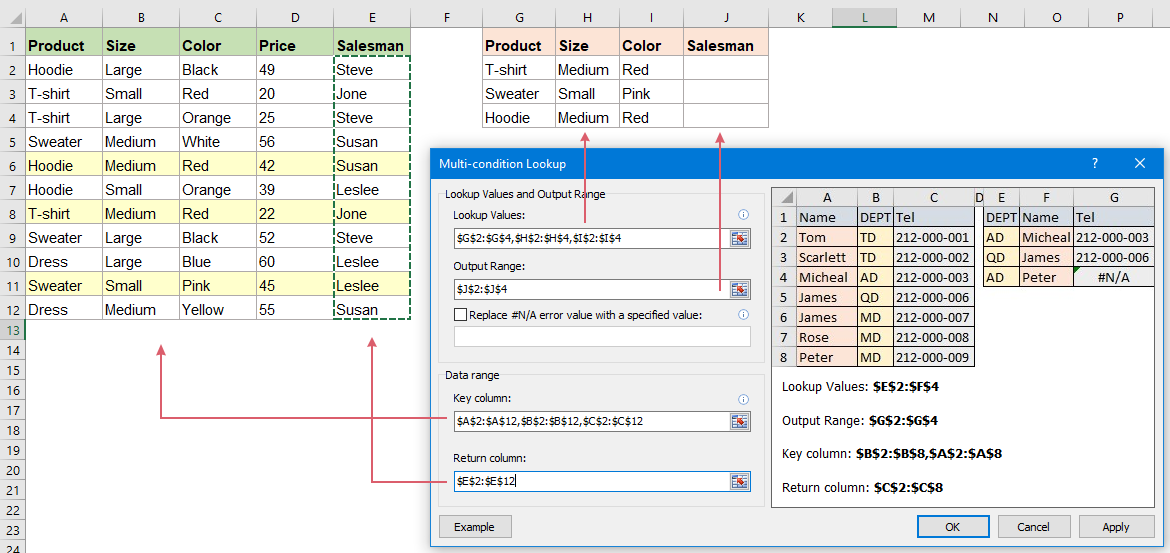3. Then, click OK or Apply button, all the matched values based on the multiple criteria have been extracted at once, see screenshot:#### More relative articles:

• Vlookup Values Across Multiple Worksheets
• In excel, we can easily apply the vlookup function to return the matching values in a single table of a worksheet. But, have you ever considered that how to vlookup value across multiple worksheet? Supposing I have the following three worksheets with range of data, and now, I want to get part of the corresponding values based on the criteria from these three worksheets, how to solve this job in Excel?
• Vlookup To Return Blank Or Specific Value Instead Of 0 Or N/A In Excel
• Normally, when you apply the vlookup function to return the corresponding value, if your matching cell is blank, it will return 0, and if your matching value is not found, you will get an error #N/A value as below screenshot shown. Instead of displaying the 0 or #N/A value, how can you make it show blank cell or other specific text value?
• Vlookup And Return Matching Data Between Two Values
• In Excel, we can apply the normal Vlookup function to get the corresponding value based on a given data. But, sometimes, we want to vlookup and return the matching value between two values as the following screenshot shown, how could you deal with this task in Excel?
• Vlookup And Return Whole / Entire Row Of A Matched Value
• Normally, you can vlookup and return a matching value from a range of data by using the Vlookup function, but, have you ever tried to find and return the whole row of data based on specific criteria as following screenshot shown.

### Best Office Productivity Tools

 Popular Features: Find, Highlight or Identify Duplicates   |  Delete Blank Rows   |  Combine Columns or Cells without Losing Data   |   Round without Formula ... Super Lookup: Multiple Criteria VLookup  |   Multiple Value VLookup  |   VLookup Across Multiple Sheets   |   Fuzzy Lookup .... Advanced Drop-down List: Quickly Create Drop Down List   |  Dependent Drop Down List   |  Multi-select Drop Down List .... Column Manager: Add a Specific Number of Columns  |  Move Columns  |  Toggle Visibility Status of Hidden Columns  |  Compare Ranges & Columns ... Featured Features: Grid Focus   |  Design View   |   Big Formula Bar   |  Workbook & Sheet Manager   |  Resource Library (Auto Text)   |  Date Picker   |  Combine Worksheets   |  Encrypt/Decrypt Cells   |  Send Emails by List   |  Super Filter   |   Special Filter (filter bold/italic/strikethrough...) ... Top 15 Toolsets:  12 Text Tools (Add Text, Remove Characters, ...)   |   50+ Chart Types (Gantt Chart, ...)   |   40+ Practical Formulas (Calculate age based on birthday, ...)   |   19 Insertion Tools (Insert QR Code, Insert Picture from Path, ...)   |   12 Conversion Tools (Numbers to Words, Currency Conversion, ...)   |   7 Merge & Split Tools (Advanced Combine Rows, Split Cells, ...)   |   ... and more

Supercharge Your Excel Skills with Kutools for Excel, and Experience Efficiency Like Never Before. Kutools for Excel Offers Over 300 Advanced Features to Boost Productivity and Save Time.  Click Here to Get The Feature You Need The Most...#### Office Tab Brings Tabbed interface to Office, and Make Your Work Much Easier

• Enable tabbed editing and reading in Word, Excel, PowerPoint, Publisher, Access, Visio and Project.
• Open and create multiple documents in new tabs of the same window, rather than in new windows.
• Increases your productivity by 50%, and reduces hundreds of mouse clicks for you every day!No ratings yet. Be the first to rate!
This comment was minimized by the moderator on the site
2,1 itu fungsinya apa yah?
This comment was minimized by the moderator on the site
I have sheet where 2 values should be verify from table available in another file in which 2 values from sheet are common and after matching both the criteria e.g Size and type from table it should capture price
This comment was minimized by the moderator on the site
Hello excelmaster,
How are you? You can lookup values in another file. Let me show you two ways.
Solution 1:
In photo 1,  sheet1 has the original data of the product details. In photo 2, we need to know the price of some items. We can use the help of the new Excel XLOOKUP function to do the trick.The syntax is =XLOOKUP(lookup_value, lookup_array, return_array, [if_not_found], [match_mode], [search_mode]).Omitting the optional arguments, =XLOOKUP(lookup_value, lookup_array, return_array)In cell E2 of sheet2, please input the formula: =XLOOKUP(A2&B2&C2,Sheet1!A2:A12&Sheet1!B2:B12&Sheet1!C2:C12,Sheet1!D2:D12)Then you get the price of the item in E2. To get the rest of the result, we need to keep the arrays in E2 formula absolute.Then the formula becomes:=XLOOKUP(A2&B2&C2,Sheet1!\$A\$2:\$A\$12&Sheet1!\$B\$2:\$B\$12&Sheet1!\$C\$2:\$C\$12,Sheet1!\$D\$2:\$D\$12)
Then drag the autofill handle down to get the rest of the results.
Solution 2:Use the Multi-condition Lookup feature in Kutools for Excel. All results will be returned at the same time.Please see photo 3, set the values in Multi-condition Lookup dialog box. Click the OK button to get the results.Please see photo 4, results in sheet2 are returned based on the data in sheet1.
Sincerely,Mandy
This comment was minimized by the moderator on the site
How do i do this
100 100100 ABC100101 DEF101102103 HIJ103
Results i want
100 ABC
100 ABC
100 ABC
100 ABC
101 DEF
101 DEF
102
103 HIJ
103 HIJ

what formula should i be using?
Thanks
This comment was minimized by the moderator on the site
The lookup will not work if there is a formula in the cell, what is the remedy ??
This comment was minimized by the moderator on the site
you are too genius, you solve my issue.
This comment was minimized by the moderator on the site
This is an elegant formula, also easily expansible to more criteria. The one donwside of INDEX+MATCH formulas is that it's really slow in larger datasets.
This comment was minimized by the moderator on the site
Index match should be faster in my personal opinion. It has been tested as well by many. If uses index match in an array, definitely it will be slower since it will become like a volatile formula. The above formula uses index match in array for multiple criteria condition which actually can be change to non-array type as well ;)
This comment was minimized by the moderator on the site
Thanks for this tutorial; :-) I have a question. What formula should I used? I have a series of data in a row like A1:M1, I'd like a result that if there is/are data that is/are < or > in specific number, it will result to "Disqualified" if it's true or " " (space) if false.
This comment was minimized by the moderator on the site
Hello, Thanks for this tutorial, it's very helpful. The following formula works great. =LOOKUP(2,1/(B:B=H97)/(I:I=H98),E:E). I have a simple question. What I want is, the cell should get the value if (H98 = open) If "open" is not there in (I:I) match (H99 = Under observation) from (I:I) and get the value, If possible get the row. I want to keep the formula as lite as possible. As I will be copying this formula in lots of cells. Also kindly suggest which of the above formula (LOOKUP/SUMPRODUCT/INDEX) is less processor intensive.
This comment was minimized by the moderator on the site
=LOOKUP(2,1/(A2:A10=G2)/(B2:B10=G3),(D2:D10)) what does the 2 mean?
There are no comments posted here yet